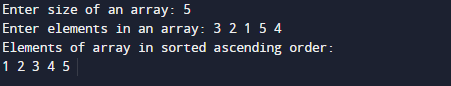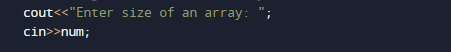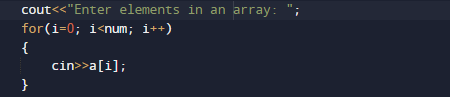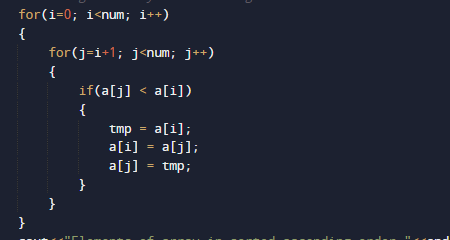# C++ Programs to Sort Array Element

In this tutorial you will learn about the C++ Programs to Sort Array Element and its application with practical example.

In this tutorial, we will learn to create a C++ program that will sort Array Elements using C++ programming.

## Prerequisites

Before starting with this tutorial we assume that you are best aware of the following C++ programming topics:

• Operators.
• looping statements.
• Basic input/output.
• Basic c++ programming
• For Loop.
• Array.

## What is array?

An Array is a collection variable elements  that can store multiple values in a single variable of same type.In Computer Science,an array is a data structure, or simply an array, is a data structure consisting of a collection of elements.

## C++ Programs to Sort Array Element.

In this program we will sort  Array elements. We would first declared and initialized the required variables. then  we would prompt user to input elements of array .Later we sort  an Array elements in ascending order.

## OutputIn the above program, we have first declared and initialized a set variables required in the program.

• a[] = it will hold entered elements.
• num =it will hold size of array.
• i and j= for iteration.
• tmp= it hold temporary values.

In our program, we will take 5 values form user in an array , after taking these values we will sort the elements of an array.
First of all we  take size of an array from user in variable num.After taking size of an array user will prompted to enter the elements of array.Here  we will use selection sort algorithm sorts an array by repeatedly finding the minimum element from unsorted part and putting it at the beginning.In every iteration sorted value is arranging in a[i] .At the end the value of sorted array will be printed.In this tutorial we have learn about the C++ Programs to Sort Array Element and its application with practical example. I hope you will like this tutorial.